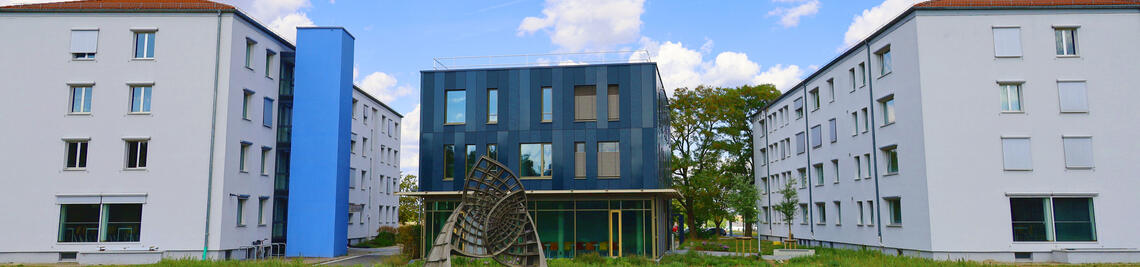•# Oberseminar Deformationsquantisierung und Geometrie: Multiplicative frame bundle of a Lie/VB groupoid'' by Francesco Cattafi (JMU Würzburg)

 Datum: 24.11.2023, 14:00 Uhr Kategorie: Veranstaltung

Abstract: It is well known that the collection of linear frames of a smooth $n$-manifold $M$ defines a principal $GL(n,\mathbb{R})$-bundle over $M$ (called the frame bundle); more generally, this construction makes sense for any vector bundle over $M$. Conversely, any principal bundle together with a representation induces an associated vector bundle; these processes establish therefore a correspondence between vector bundles on one side, and principal bundles with representations on the other side.

If instead of a manifold $M$ we begin with a Lie groupoid $\mathcal{G} \rightrightarrows M$, one can consider both the frame bundles of $\mathcal{G}$ and of $M$ and try to "close" the resulting diagram in a natural way. The frame bundle of $\mathcal{G}$ is however too big to support a Lie groupoid structure over the frame bundle of $M$. In this talk, I will discuss how to fix this issue by introducing a special class of frames which interact nicely with the groupoid structure ("multiplicative frames"). At the end, I will sketch how to generalise this construction to a correspondence between VB-groupoids (groupoid objects in the category of vector bundles) and PB-groupoids (groupoid objects in the category of principal bundles). This is a joint work with Alfonso Garmendia.